Courses

# Ex 6.2 NCERT Solutions- Triangles Class 10 Notes | EduRev

## Class 10 : Ex 6.2 NCERT Solutions- Triangles Class 10 Notes | EduRev

The document Ex 6.2 NCERT Solutions- Triangles Class 10 Notes | EduRev is a part of the Class 10 Course Mathematics (Maths) Class 10.
All you need of Class 10 at this link: Class 10

Exercise 6.2
Q.1. In figures (i) and (ii), DE y BC. Find EC in (i) and AD in (ii).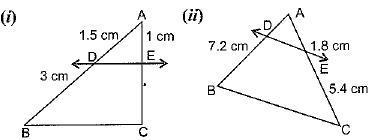Sol.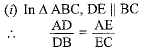[By basic proportional theorem]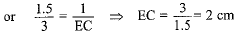(ii) In ΔABC, DE || BC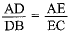[By basic proportional theorem]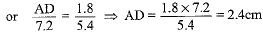Q.2. E and F are points on the sides PQ and PR respectively of a ΔPQR. For each of the following cases, state whether EF || QR:
(i) PE = 3.9 cm, EQ = 3 cm, PF = 3.6 cm and FR = 2.4 cm
(ii) PE = 4 cm, QE = 4.5 cm, PF = 8 cm and RF = 9 cm
(iii) PQ = 1.28 cm, PR = 2.56 cm, PE = 0.18 cm and PF = 0.36 cm
Sol. (i)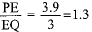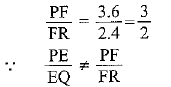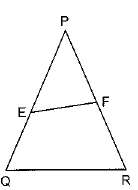⇒ EF is not parallel to QR
[By converse of B.P.T.]
(ii)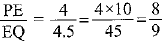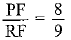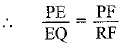⇒ EF || QR [By converse of B.P.T.]
(iii)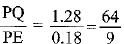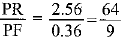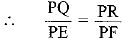⇒ EF || QR [By converse of B.P.T.]

Q.3. In the figure, if LM || CB and LN || CD, prove that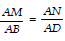.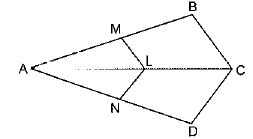Sol.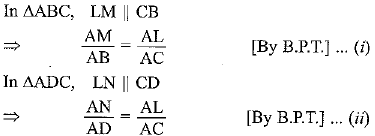From equation (i) and (ii)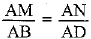Q.4. In the figure, DE || AC and DF || AE. Prove that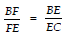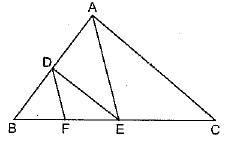Sol. In ΔABC,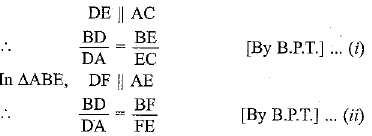From equation (i) and (ii)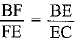Q.5. In the figure, DE || OQ and DF || OR. Show that EF || QR.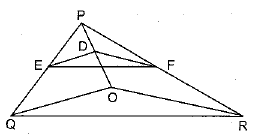Sol. In Δ PQO,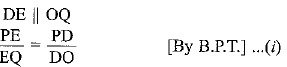In ΔPOR, DF || OR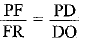...(ii)
From equation (i) and (ii), we get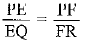∴  EF || QR [By converse of B.P.T.]

Q.6. In the figure, A, B and C are points on OP, OQ and OR respectively such that AB || PQ and AC || PR. Show that BC || QR.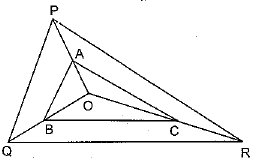Sol. In Δ PQR,
AB || PQ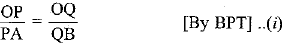In ΔPOR, AC || PR
∴  OP/PA = OR/RC    .......(ii)

From equation (i) and (ii), we get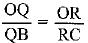BC || QR [By converse of B.P.T.]

Q.7. Using Theorem 6.1, prove that a line drawn through the mid-point of one side of a triangle parallel to another side bisects the third side.
Sol. Given: In Δ ABC, D is the mid-point of AB and DE || BC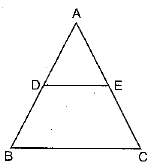To Prove: AE = EC
Proof: In ΔABC,
DE || BC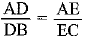[By B.P.T.]
⇒ 1 = AE/EC ⇒ AE = EC
Hence, DE bisects AC.

Q.8. Using Theorem 6.2, prove that the line joining the mid-points of any two sides of a triangle is parallel to the third side.
Sol. Given. A ΔABC in which D and E are mid-points of sides AB and AC respectively.
To Prove: DE || BC
Proof: In ΔABC, AD = DB and AE = EC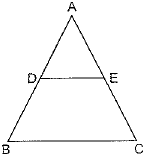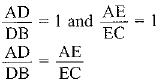∴ DE || BC   [By converse of B.P.T.]

Q.9. ABCD is a trapezium in which AB || DC and its diagonals intersect each other at the point O. Show that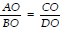.
Sol.
Given: ABCD is a trapezium in which AB || DC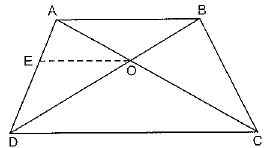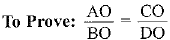Construction: Draw EO || DC
Proof: In ΔABD, EO || DC     [By construction]
DC || AB     [Given]
⇒  EO || AB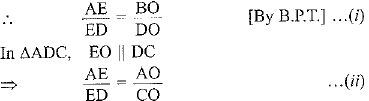From equation (i) and (ii)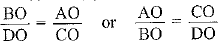Q.10. The diagonals of a quadrilateral ABCD intersect each other at the point O such that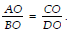. Show that ABCD is a trapezium.
Sol. Given: A quadrilateral ABCD, whose diagonals intersect at O.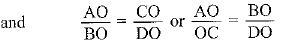To Prove: ABCD is a trapezium.
Construction: Draw EO || AB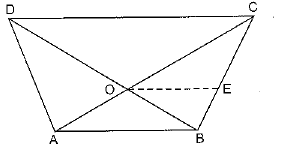Proof: In ΔABC, OE || AB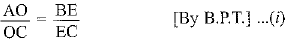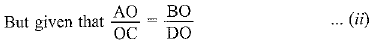From equation (i) and (ii)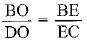⇒ OE || DC [By converse of B.P.T.]
OE || AB and OE || DC ⇒ AB || DC
∴ ABCD is a trapezium.

Offer running on EduRev: Apply code STAYHOME200 to get INR 200 off on our premium plan EduRev Infinity!

## Mathematics (Maths) Class 10

62 videos|346 docs|103 tests

,

,

,

,

,

,

,

,

,

,

,

,

,

,

,

,

,

,

,

,

,

;